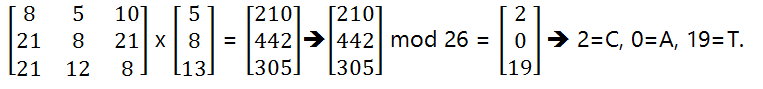# Cryptographic Techniques: Vigenére Cipher, Playfair Cipher, Hill Cipher

In this article, we are going to learn three Cryptography Techniques: Vigenére Cipher, Playfair Cipher, and Hill Cipher.
Submitted by Himanshu Bhatt, on September 22, 2018

## 1) Vigenére Cipher

This technique is an example of Polyalphabetic Substitution technique which uses 26 Caesar ciphers make up the mono-alphabetic substitution rules which follow a count shifting mechanism from 0 to 25. That is, for each plain-text character we have a corresponding substitution known as a key letter.

To understand this technique we need to take a look on this Vigenére tableau.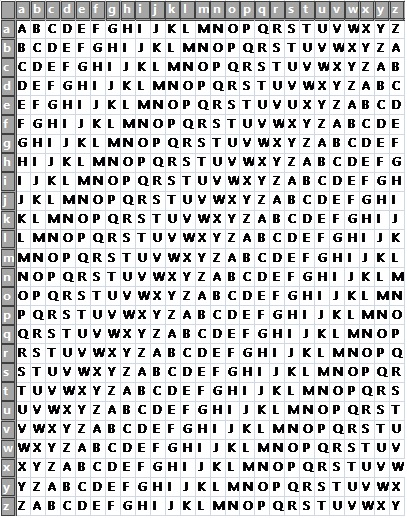The logic for this technique is simple, say for a key letter 't' and plain-text letter 'h', the corresponding cipher-text letter is at the intersection of row titled 't' and column titled 'h' thus for this case we get a ciphertext that would be 'A' based on above table.

It is clear that for encrypting a plain-text message we must need a key whose length is equal to the size of the plain-text message usually a repeating key is used.

## 2) Playfair Cipher

Playfair cipher (aka Playfair square) is a cryptography technique used for manual encryption, it was introduced by Charles Wheatstone in 1854 but it was popularized by Lord Playfair hence his name used.

The Playfair cipher was used in world war I by the British army and in World war II it was used by Australians. It was because the Playfair cipher is quite fast to use and does not demand any special equipment.

Playfair technique has 2 major steps: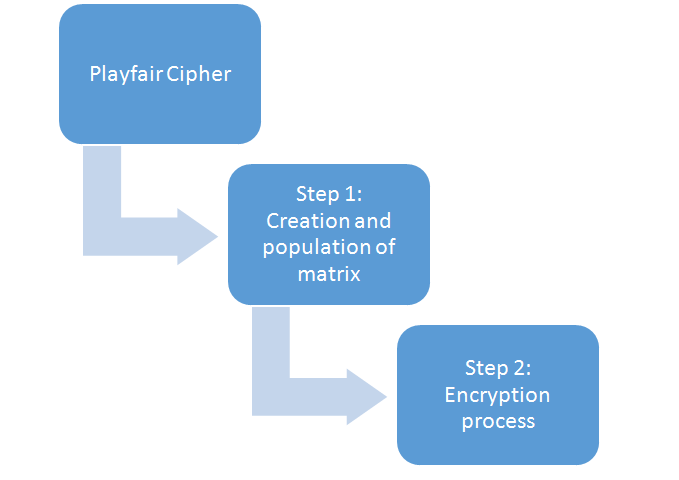Step 1: Creation and Population of matrix

This technique uses a 5 x 5 matrix which stores a keyword (or phrase) that becomes the key for encryption or decryption. This involves some set of fixed rule, those are:

1. Fill the keyword in the matrix row-wise from left to right, top to down.
2. Remove all repeated letters.
3. Enter the remaining spaces in the matrix with the rest of the English alphabets (A-Z) that were not a part of our keyword. In this process, combine I and J in the same cell of the table; in other words, if I and J is a part of the keyword disregard both I and J while filling the remaining slots.

Example:

Let's take our keyword as 'INCLUDEHELP IS AWESOME'. Then 5 x 5 matrix will look as: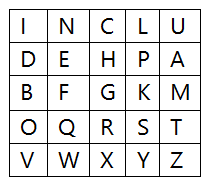Step 2: Encryption

This process consist of the following process:

1. The plain-text we want to encrypt need to broke down into a pair of alphabets. For example, if our message is "MY NAME IS POGO", it will become "MY NA ME IS PO GO".
2. If both alphabets are the same (or only left), add an X after the first alphabet in a pair. Encrypt the new pair (after adding X) then continue.
1. If pair appears in the same row of the matrix, replace them with a letter at its right, if it's at the right end of the row then wrapping around the left side of the row.
3. If pair appears in the same column of the matrix, replace them with the alphabet below them. If it's on the bottom then wrapping around the top of the row.
4. If the pairis not in the same row or column, replace them with the alphabets in the same row respectively, but at the other pair of cornersof the rectangle defined by the original pair. The order is quite significant here. The first encrypt alphabet of the pair is the one that is present on the same row as the first plaintext alphabet.

Explanation:

"MY NA ME IS PO GO"

So we start with MY now in the above matrix it was not in the same row or column then rule 5 will apply.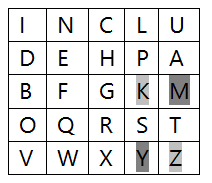Now we replace 'MY' with the diagonals that are 'KZ'.

Let's move to 'NA' again they are not in the same row or column, rule 5 will apply.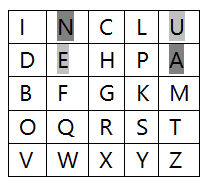Now, 'NA' will be replaced with 'UE'.

Similarly, "MY NA ME IS PO GO" will become "KZ UE FA LO DS BS".

For the decryptionprocess, we will just follow the exact opposite steps.

## 3) Hill Cipher

The Hill cipher is based on polygraphic substitution that is it works on multiple letters at the same time, introduced by Lester Hill in 1929. This method related to the matrix theory of mathematics, specifically how to compute the inverse of a matrix.

Let's see how it works:

1. Every Letter of plain-text will be represented by a number, so that A=0, B=1,...,Z=25.
2. We will make a matrix of the plain-text message using the above knowledge.
For example, 'CAT', so C=2, A=0, T=19. Thus our matrix lookslike: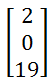3. Now, we multiply our matrix with randomly chosen keys. The size of the key must be n x n where n is a number of rows of our plain-text matrix.
For example: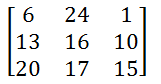4. Now multiply the above matrices,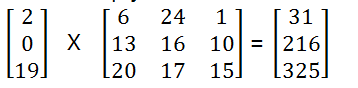5. Now we will calculate a mod 26 value of the above matrix, that is remainder after dividing 26.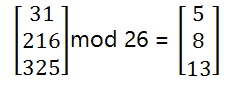6. Translate back number to alphabets, 5=F, 8=I, 13=N. Therefore, our cipher-text is "FIN".
7. For Decryption, take the cipher-text matrix and multiply it with the inverse of our original key matrix: